# Injective module

(diff) ← Older revision | Latest revision (diff) | Newer revision → (diff)
Jump to: navigation, search

An injective object in the category of (right) modules over an associative ring with identity, i.e. an-modulesuch that for any-modules,, for any monomorphism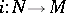, and for any homomorphism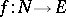there is a homomorphismthat makes the following diagram commutative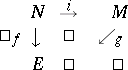Here and below all-modules are supposed to be right-modules. The following conditions on an-moduleare equivalent to injectivity: 1) for any exact sequence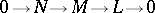the induced sequenceis exact; 2) any exact sequence of-modules of the formsplits, i.e. the submoduleis a direct summand of; 3)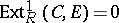for all-modules; and 4) for any right idealofa homomorphism of-modulescan be extended to a homomorphism of-modules(Baer's criterion). There are "enough" injective objects in the category of-modules: Each-modulecan be imbedded in an injective module. Moreover, each modulehas an injective hull, i.e. an injective module containingin such a way that each non-zero submodule ofhas non-empty intersection with. Any imbedding of a moduleinto an injective modulecan be extended to an imbedding ofinto. Every-modulehas an injective resolution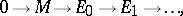i.e. an exact sequence of modules in which each module,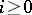, is injective. The length of the shortest injective resolution is called the injective dimension of the module (cf. also Homological dimension).

A direct product of injective modules is an injective module. An injective moduleis equal to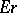for any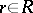that is not a left zero divisor in, i.e. an injective module is divisible. In particular, an Abelian group is an injective module over the ring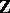if and only if it is divisible. Letbe a commutative Noetherian ring. Then any injective module over it is a direct sum of injective hulls of modules of the form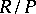, whereis a prime ideal in.

Injective modules are extensively used in the description of various classes of rings (cf. Homological classification of rings). Thus, all modules over a ring are injective if and only if the ring is semi-simple. The following conditions are equivalent:is a right Noetherian ring; any direct sum of injective-modules is injective; any injective-module is decomposable as a direct sum of indecomposable-modules. A ringis right Artinian if and only if every injective module is a direct sum of injective hulls of simple modules. A ringis right hereditary if and only if all its quotient modules by injective-modules are injective, and also if and only if the sum of two injective submodules of an arbitrary-module is injective. If the ringis right hereditary and right Noetherian, then every-module contains a largest injective submodule. The projectivity (injectivity) of all injective (projective)-modules is equivalent tobeing a quasi-Frobenius ring.

The injective hull of the moduleplays an important role in the theory of rings of fractions. E.g., if the right singular ideal of a ringvanishes, ifis the injective hull of the module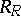, and ifis its endomorphism ring, then the-modulesandare isomorphic,is a ring isomorphic to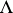and is also the maximal right ring of fractions of, and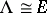is a self-injective right regular ring (in the sense of von Neumann).

In connection with various problems on extending module homomorphisms, some classes of modulesclose to injective modules have been considered: quasi-injective modules (ifand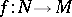, thencan be extended to an endomorphism of); pseudo-injective modules (if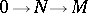andis a monomorphism, thencan be extended to an endomorphism of); and small-injective modules (all endomorphisms of submodules can be extended to endomorphisms of). The quasi-injectivity of a moduleis equivalent to the invariance ofin its injective hull under endomorphisms of the latter.

How to Cite This Entry:
Injective module. Encyclopedia of Mathematics. URL: http://encyclopediaofmath.org/index.php?title=Injective_module&oldid=16793
This article was adapted from an original article by A.V. MikhalevA.A. Tuganbaev (originator), which appeared in Encyclopedia of Mathematics - ISBN 1402006098. See original article